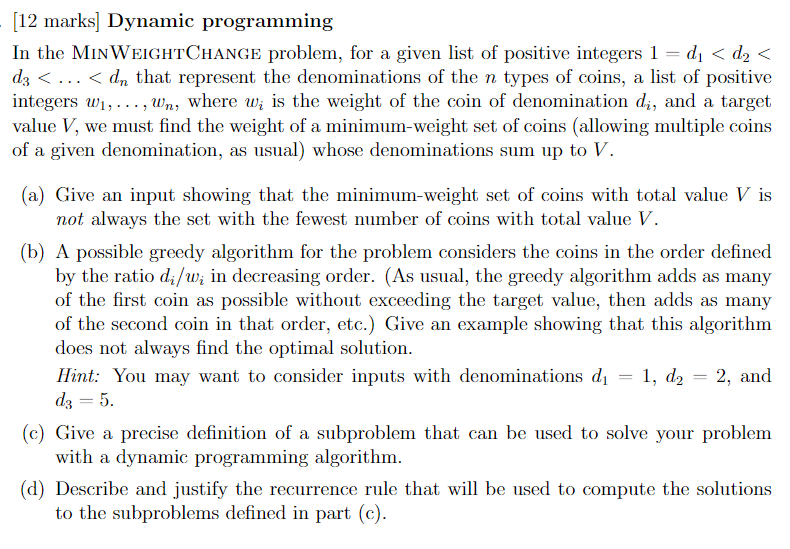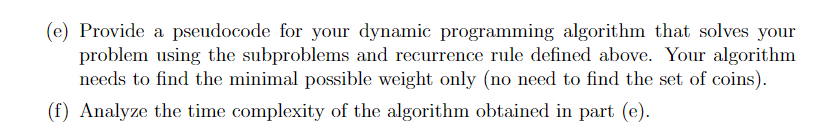# Question [12 marks] Dynamic programming In the MinWeightChange problem, for a given list of positive integers $$1=d_{1}<d_{2}<$$ $$d_{3}<\ldots<d_{n}$$ that represent the denominations of the $$n$$ types of coins, a list of positive integers $$w_{1}, \ldots, w_{n}$$, where $$w_{i}$$ is the weight of the coin of denomination $$d_{i}$$, and a target value $$V$$, we must find the weight of a minimum-weight set of coins (allowing multiple coins of a given denomination, as usual) whose denominations sum up to $$V$$. (a) Give an input showing that the minimum-weight set of coins with total value $$V$$ is not always the set with the fewest number of coins with total value $$V$$. (b) A possible greedy algorithm for the problem considers the coins in the order defined by the ratio $$d_{i} / w_{i}$$ in decreasing order. (As usual, the greedy algorithm adds as many of the first coin as possible without exceeding the target value, then adds as many of the second coin in that order, etc.) Give an example showing that this algorithm does not always find the optimal solution. Hint: You may want to consider inputs with denominations $$d_{1}=1, d_{2}=2$$, and $$d_{3}=5$$ (c) Give a precise definition of a subproblem that can be used to solve your problem with a dynamic programming algorithm. (d) Describe and justify the recurrence rule that will be used to compute the solutions to the subproblems defined in part (c). (e) Provide a pseudocode for your dynamic programming algorithm that solves your problem using the subproblems and recurrence rule defined above. Your algorithm needs to find the minimal possible weight only (no need to find the set of coins). (f) Analyze the time complexity of the algorithm obtained in part (e).UAEMEH The Asker · Computer ScienceTranscribed Image Text: [12 marks] Dynamic programming In the MinWeightChange problem, for a given list of positive integers $$1=d_{1} More Transcribed Image Text: [12 marks] Dynamic programming In the MinWeightChange problem, for a given list of positive integers \( 1=d_{1} Community AnswerKV6TCL &#12304;General guidance&#12305;The answer provided below has been developed in a clear step by step manner.Step1/2Below are the solutions for all subparts from a to f:(a) Example: Let the denominations be d&#8321;=1, d&#8322;=3, d&#8323;=4, and the weights be w&#8321;=3, w&#8322;=2, w&#8323;=1, and the target value be V=6. Explanation:The optimal solution would be to use two coins of denomination 3, with a total weight of 4. However, using two coins of denomination 1 and two coins of denomination 2 would also sum up to 6 but have a total weight of 8.(b) Example: Let the denominations be d&#8321;=1, d&#8322;=2, and d&#8323;=d&#8324;=5, and the weights be w&#8321;=1, w&#8322;=2, w&#8323;=5, w&#8324;=10, and the target value be V=12. Explanation:The greedy algorithm would first use two coins of denomination 5, with a total weight of 10. Then, it would add two coins of denomination 1, with a total weight of 2, resulting in a total weight of 12. However, a better solution would be to use one coin of denomination 5 and one coin of denomination 2, with a total weight of 3.(c) A subproblem can be defined as finding the minimum weight of a set of coins that sum up to a value less than or equal to some target value k, using only the first i denominations of coins.(d) Let \( \mathrm{{M}{\left({i},{k}\right)}}$$be the minimum weight of a set of coins that sum up to a value less than or equal to k, using only the first $$\mathrm{{i}}$$ denominations of coins. Then the recurrence rule is:$$\mathrm{{M}{\left({i},{k}\right)}=\min{\left\lbrace{M}{\left({i}-{1},{k}\right)},{w}_{{i}}+{M}{\left({i},{k}-{d}_{{i}}\right)}\right\rbrace}}$$Explanation:Please refer to solution in this step.Step2/2(e) Pseudocode for dynamic programming algorithm: ... See the full answer